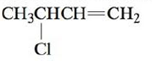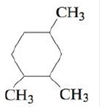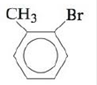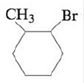# Name each of the following compounds. a. b. c. d. e. f. g.### Chemistry: An Atoms First Approach

2nd Edition
Steven S. Zumdahl + 1 other
Publisher: Cengage Learning
ISBN: 9781305079243

#### Solutions

Chapter
Section### Chemistry: An Atoms First Approach

2nd Edition
Steven S. Zumdahl + 1 other
Publisher: Cengage Learning
ISBN: 9781305079243
Chapter 21, Problem 32E
Textbook Problem
1 views

## Name each of the following compounds.a.b.c.d.e.f.g.(a)

Interpretation Introduction

Interpretation: The given compounds are to be named.

Concept introduction: Rules given by IUPAC should be followed to name an organic compound. Any organic compound has only one name that denotes that compound. The root word determines the number of carbons while counting the longest carbon chain. If more than one substituent’s is present, prefixes like di, tri, tetra, etc. are used and different substituents are written in alphabetical order.

### Explanation of Solution

Explanation

To determine: The name of the given organic compound.

The name of the compound is 3-chlorobut-1-ene.

The given compound is,

(b)

Interpretation Introduction

Interpretation: The given compounds are to be named.

Concept introduction: Rules given by IUPAC should be followed to name an organic compound. Any organic compound has only one name that denotes that compound. The root word determines the number of carbons while counting the longest carbon chain. If more than one substituent’s is present, prefixes like di, tri, tetra, etc. are used and different substituents are written in alphabetical order.

(c)

Interpretation Introduction

Interpretation: The given compounds are to be named.

Concept introduction: Rules given by IUPAC should be followed to name an organic compound. Any organic compound has only one name that denotes that compound. The root word determines the number of carbons while counting the longest carbon chain. If more than one substituent’s is present, prefixes like di, tri, tetra, etc. are used and different substituents are written in alphabetical order.

(d)

Interpretation Introduction

Interpretation: The given compounds are to be named.

Concept introduction: Rules given by IUPAC should be followed to name an organic compound. Any organic compound has only one name that denotes that compound. The root word determines the number of carbons while counting the longest carbon chain. If more than one substituent’s is present, prefixes like di, tri, tetra, etc. are used and different substituents are written in alphabetical order.

(e)

Interpretation Introduction

Interpretation: The given compounds are to be named.

Concept introduction: Rules given by IUPAC should be followed to name an organic compound. Any organic compound has only one name that denotes that compound. The root word determines the number of carbons while counting the longest carbon chain. If more than one substituent’s is present, prefixes like di, tri, tetra, etc. are used and different substituents are written in alphabetical order.

(f)

Interpretation Introduction

Interpretation: The given compounds are to be named.

Concept introduction: Rules given by IUPAC should be followed to name an organic compound. Any organic compound has only one name that denotes that compound. The root word determines the number of carbons while counting the longest carbon chain. If more than one substituent’s is present, prefixes like di, tri, tetra, etc. are used and different substituents are written in alphabetical order.

(g)

Interpretation Introduction

Interpretation: The given compounds are to be named.

Concept introduction: Rules given by IUPAC should be followed to name an organic compound. Any organic compound has only one name that denotes that compound. The root word determines the number of carbons while counting the longest carbon chain. If more than one substituent’s is present, prefixes like di, tri, tetra, etc. are used and different substituents are written in alphabetical order.

### Still sussing out bartleby?

Check out a sample textbook solution.

See a sample solution

#### The Solution to Your Study Problems

Bartleby provides explanations to thousands of textbook problems written by our experts, many with advanced degrees!

Get Started

Find more solutions based on key concepts
Bile is essential for fat digestion because it splits triglycerides into fatty acids and glycerol. emulsifies f...

Nutrition: Concepts and Controversies - Standalone book (MindTap Course List)

Does the cell cycle refer to mitosis as well as meiosis?

Human Heredity: Principles and Issues (MindTap Course List)

In a cyclotron (one type of particle accelerator), a deuteron (of mass 2.00 u) reaches a final speed of 10.0% o...

Physics for Scientists and Engineers, Technology Update (No access codes included)

What would a standing wave look like in a rectangular swimming pool?

Oceanography: An Invitation To Marine Science, Loose-leaf Versin

Show that the integral 0e2t/RCdtin Example 27.11 has the 12RC.

Physics for Scientists and Engineers with Modern Physics